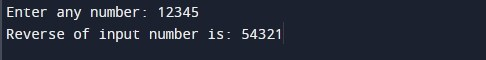C Program to Reverse Number Using While Loop and Recursion

In this tutorial you will learn about the C Program to Reverse Number Using While Loop and Recursion and its application with practical example.

In this tutorial, we will learn to create a c program to reverse number using while loop and using recursion. For example, if the input number is 123, then the output will be 321. We will show you two different method to reverse a given number:

• Using while loop
• Using recursion

Prerequisites

Before starting with this tutorial we assume that you are best aware of the following C programming topics:

• C Data Types
• C Operator
• C If else
• C while loop
• C Function
• C Recursion

C Program to reverse number using while loop

In this program we will reverse a number using while loop. We have first declared and initialized the required variables. Next, we would prompt user to input a number to be reversed. Later in the program we will reverse the number using while loop. We will then display the reversed number of the given number to user.

Output:-In the above program, we have first declared and initialized a set variables required in the program.

• num = it holds the first integer number value
• rnum = will hold reversed number, initialized with 0
• rem = it holds the remainder during operations

In the next statement user will be prompted to enter an integer number which will be assigned to variable ‘num’. Next, we will compute the reverse of the given number using c while loop. In the program, we use the modulus operator (%) to obtain individual digits of the number. Finally, we will be displaying the reversed number of the given number using printf statement.

C Program to reverse number using recursion

In this program we will reverse a number using a recursive function. A function is called recursive when calls itself. We have first defined a recursive function in the program to return reverse of the given number. Next, we have declared and initialized the required variables. We would then prompt user to input a number to be reversed. Later in the program we have invoked the reverse() function to return reverse value of the number. We will then display the reversed number of the given number.

Output:-In the above program, we have first defined a function called as reverse(). Thie reverse() function will compute the reverse of the given number. Next, we have declared required variables in the main function.

• num = it holds the first integer number value
• rnum = will hold reversed number

Next, user prompted to enter a number which assigned to variable ‘num’. Next, we will be calling reverse() function to compute and return reverse value of the ‘num’ variable. Finally, we will display the reversed number of the given number.

In this tutorial we have learn about the C Program to Reverse Number Using While Loop and Recursion and its application with practical example. I hope you will like this tutorial.